# [Solved] Rstudio Error: Error in `vec_as_location()`: `…` must be empty.

In the process of running the dplyr package installation, you may be prompted with this error, and you can install it again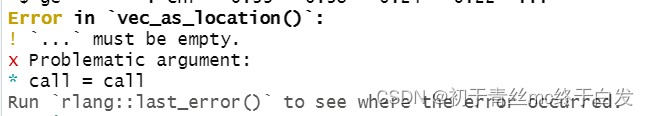``````Error in `vec_as_location()`:
! `...` must be empty.
x Problematic argument:
* call = call
Run `rlang::last_error()` to see where the error occurred.
``````

Solutions

``install.packages("dplyr")``

# [Solved] R fitdistr Error: non-finite finite-difference value

Error Message:

```par<-MASS::fitdistr(xd,“gamma”)

Error in stats::optim(x = c(275.246894836426, 344.755493164062, 4575.26025390625, :
non-finite finite-difference value ```

Solution: Scaling the data
par<-MASS::fitdistr(xd/100,“gamma”)

# [Solved] Error in Summary.factor ‘sum’ not meaningful for factors

Question:

The root cause is the wrong data type.

The factor type has no sum method

``````#create a vector of class vector
factor_vector <- as.factor(c(1, 7, 12, 14, 15))

#attempt to find min value in the vector
sum(factor_vector)

Error in Summary.factor(1:5, na.rm = FALSE) :
‘sum’ not meaningful for factors``````

Solution:
Convert to numeric values and use the as.numeric function.
mydata\$value<-as.numeric(mydata\$value)
is.numeric(mydata\$value)

``````#convert factor vector to numeric vector and find the min value
new_vector <- as.numeric(as.character(factor_vector))
sum(new_vector)

# 49``````

Complete error:

```#create a vector of class vector
factor_vector <- as.factor(c(1, 7, 12, 14, 15))

#attempt to find min value in the vector
sum(factor_vector)

Error in Summary.factor(1:5, na.rm = FALSE) :
‘sum’ not meaningful for factors```

Other (the minimum value can be obtained for numeric value, string and date type)

Numeric value, string and date type can all be maximized. Similarly, the maximum value can be obtained.

``````numeric_vector <- c(1, 2, 12, 14)
max(numeric_vector)

# 14

character_vector <- c("a", "b", "f")
max(character_vector)

# "f"

date_vector <- as.Date(c("2019-01-01", "2019-03-05", "2019-03-04"))
max(date_vector)

# "2019-03-05"``````

The R language is called R partly because of the names of the two R authors (Robert gentleman and Ross ihaka) and partly because of the influence of Bell Labs s language (called the dialect of s language).

R language is a mathematical programming language designed for mathematical researchers. It is mainly used for statistical analysis, drawing and data mining.

If you are a beginner of computer programs and are eager to understand the general programming of computers, R language is not an ideal choice. You can choose python, C or Java.

Both R language and C language are the research achievements of Bell Laboratories, but they have different emphasis areas. R language is an explanatory language for mathematical theory researchers, while C language is designed for computer software engineers.

R language is a language for interpretation and operation (different from the compilation and operation of C language). Its execution speed is much slower than that of C language, which is not conducive to optimization. However, it provides more abundant data structure operation at the syntax level and can easily output text and graphic information, so it is widely used in mathematics, especially in statistics

# [Solved] Error in Summary.factor ‘max’ not meaningful for factors

Question:

The root cause is the wrong data type.

The factor type has no max method

``````#create a vector of class vector
factor_vector <- as.factor(c(1, 7, 12, 14, 15))

#attempt to find max value in the vector
max(factor_vector)

#Error in Summary.factor(1:5, na.rm = FALSE) :
#  'max' not meaningful for factors``````

Solution:

Convert to numeric value or string, here convert to numeric value.

mydata\$value<-as.numeric(mydata\$value)
is.numeric(mydata\$value)

``````#convert factor vector to numeric vector and find the max value
new_vector <- as.numeric(as.character(factor_vector))
max(new_vector)

# 15``````

Full error:

```#create a vector of class vector
factor_vector <- as.factor(c(1, 7, 12, 14, 15))

#attempt to find max value in the vector
max(factor_vector)

#Error in Summary.factor(1:5, na.rm = FALSE) :
#  'max' not meaningful for factors```

Other (numeric value, string, date type can be the maximum value)

Numeric value, string and date type can all be the maximum value, and similarly, the minimum value can be obtained.

``````numeric_vector <- c(1, 2, 12, 14)
max(numeric_vector)

# 14

character_vector <- c("a", "b", "f")
max(character_vector)

# "f"

date_vector <- as.Date(c("2019-01-01", "2019-03-05", "2019-03-04"))
max(date_vector)

# "2019-03-05"``````

# [Solved] R Error: ERROR: configuration failed for package ‘magick’

An error occurred when installing the spatialexperiment package in R

ERROR: configuration failed for package ‘magick’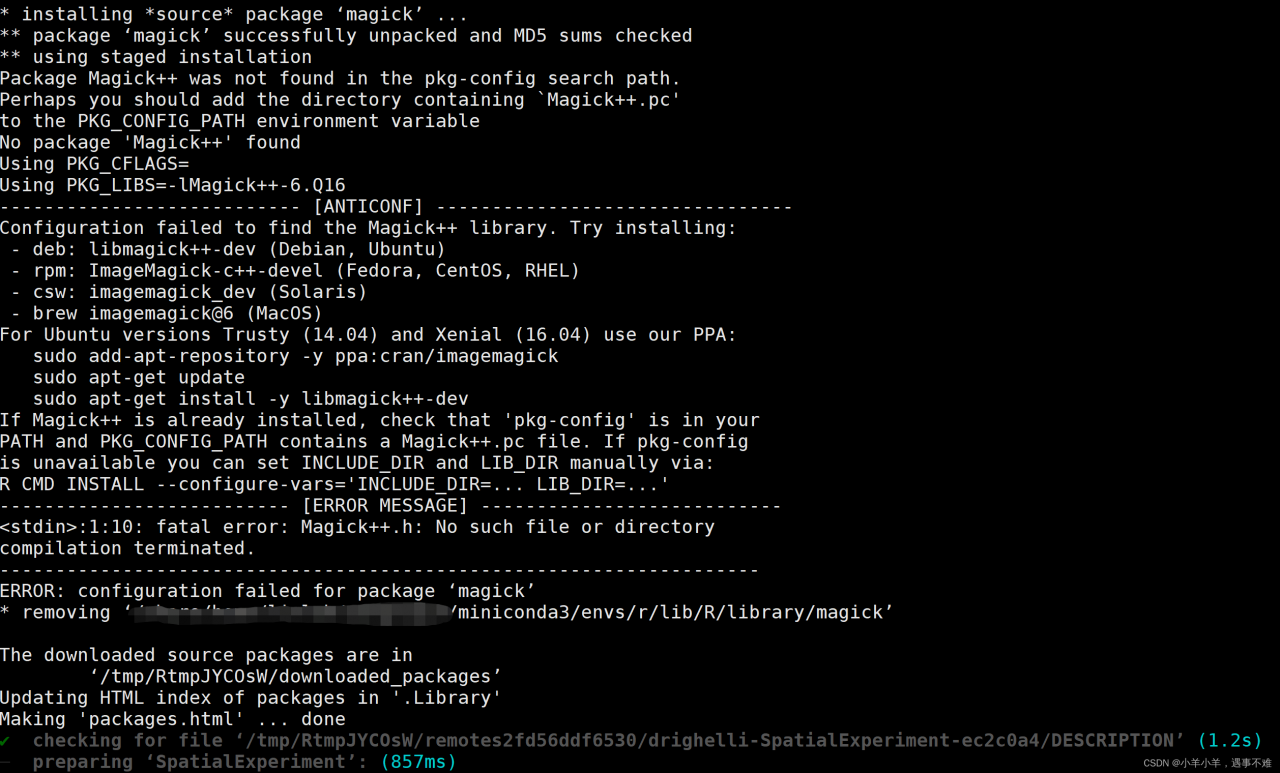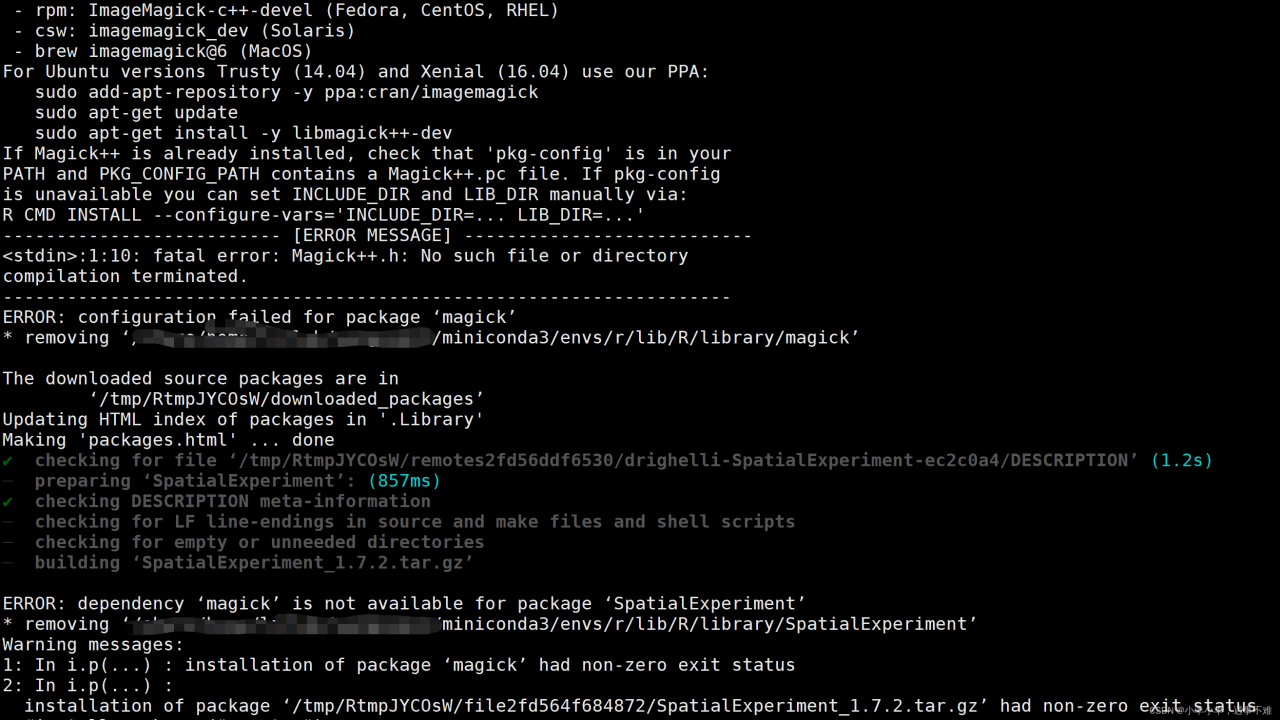The error message appears to be missing ImageMagick

Solution: try to install with conda

conda install -c conda-forge imagemagick

Done!

# R language Use setwd() function Error [How to Solve]

When using R language, an error may appear: unable to set the working directory

At this time, you can manually switch in rstudio: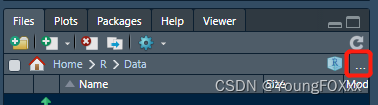Workspace window, files → three dots, click; The folder window will appear. At this time, manually switch to the working directory you want to go to: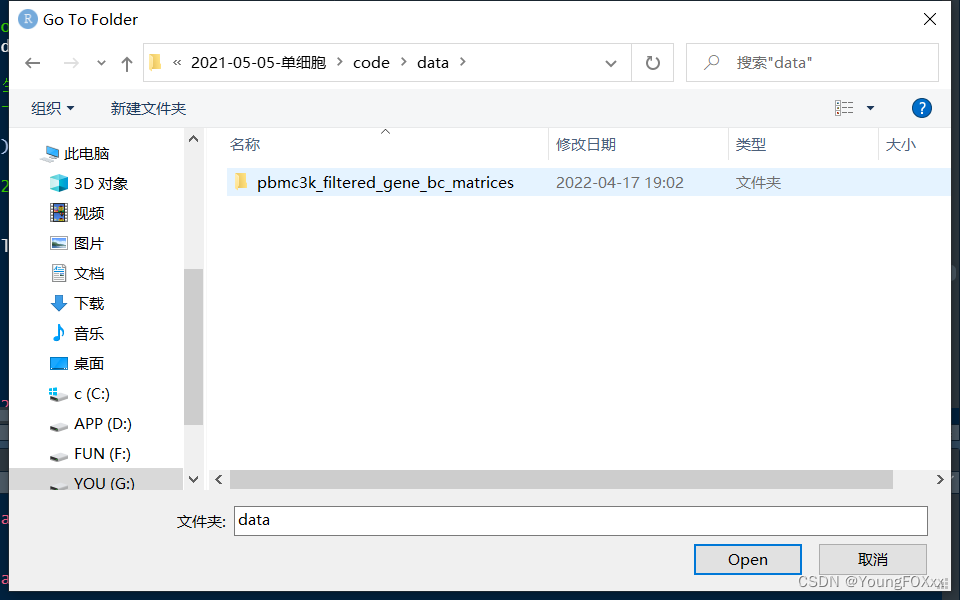Click the setting icon and select “set as working directory” to set the working directory: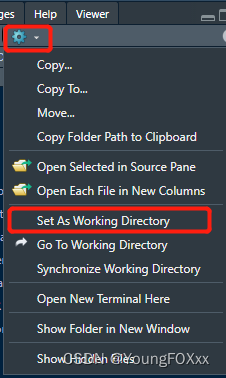At this time, the working directory is detected and the switching is successful

``````getwd()
 ".../Documents/R/Data"``````

# [Solved] Error: ‘attrition‘ is not an exported object from ‘namespace:rsample‘

Error: ‘attrition’ is not an exported object from ‘namespace:rsample’

# Import package and library

``````# load required packages
library(rsample)
library(dplyr)
library(h2o)
library(DALEX)

# initialize h2o session
h2o.no_progress()
h2o.init()
##  Connection successful!
##
## R is connected to the H2O cluster:
##     H2O cluster uptime:         4 hours 30 minutes
##     H2O cluster timezone:       America/New_York
##     H2O data parsing timezone:  UTC
##     H2O cluster version:        3.18.0.11
##     H2O cluster version age:    1 month and 17 days
##     H2O cluster total nodes:    1
##     H2O cluster total memory:   1.01 GB
##     H2O cluster total cores:    4
##     H2O cluster allowed cores:  4
##     H2O cluster healthy:        TRUE
##     H2O Connection ip:          localhost
##     H2O Connection port:        54321
##     H2O Connection proxy:       NA
##     H2O Internal Security:      FALSE
##     H2O API Extensions:         XGBoost, Algos, AutoML, Core V3, Core V4
##     R Version:                  R version 3.5.0 (2018-04-23)``````

#Data preprocessing and processing to h2o format;

Error: ‘attrition’ is not an exported object from ‘namespace:rsample’

``````#

# classification data
df <- rsample::attrition %>%
mutate_if(is.ordered, factor, ordered = FALSE) %>%
mutate(Attrition = recode(Attrition, "Yes" = "1", "No" = "0") %>% factor(levels = c("1", "0")))

# convert to h2o object
df.h2o <- as.h2o(df)

# create train, validation, and test splits
set.seed(123)
splits <- h2o.splitFrame(df.h2o, ratios = c(.7, .15), destination_frames = c("train","valid","test"))
names(splits) <- c("train","valid","test")

# variable names for resonse & features
y <- "Attrition"
x <- setdiff(names(df), y) ``````

Solution:

You can use the attrition dataset of DALEX package directly;

Remove resample:

``````#

# classification data
df <- attrition %>%
mutate_if(is.ordered, factor, ordered = FALSE) %>%
mutate(Attrition = recode(Attrition, "Yes" = "1", "No" = "0") %>% factor(levels = c("1", "0")))

# convert to h2o object
df.h2o <- as.h2o(df)

# create train, validation, and test splits
set.seed(123)
splits <- h2o.splitFrame(df.h2o, ratios = c(.7, .15), destination_frames = c("train","valid","test"))
names(splits) <- c("train","valid","test")

# variable names for resonse & features
y <- "Attrition"
x <- setdiff(names(df), y) ``````

Full Error Messages:

```> # classification data
> df <- rsample::attrition %>%
+     mutate_if(is.ordered, factor, ordered = FALSE) %>%
+     mutate(Attrition = recode(Attrition, "Yes" = "1", "No" = "0") %>% factor(levels = c("1", "0")))
Error: 'attrition' is not an exported object from 'namespace:rsample'
>```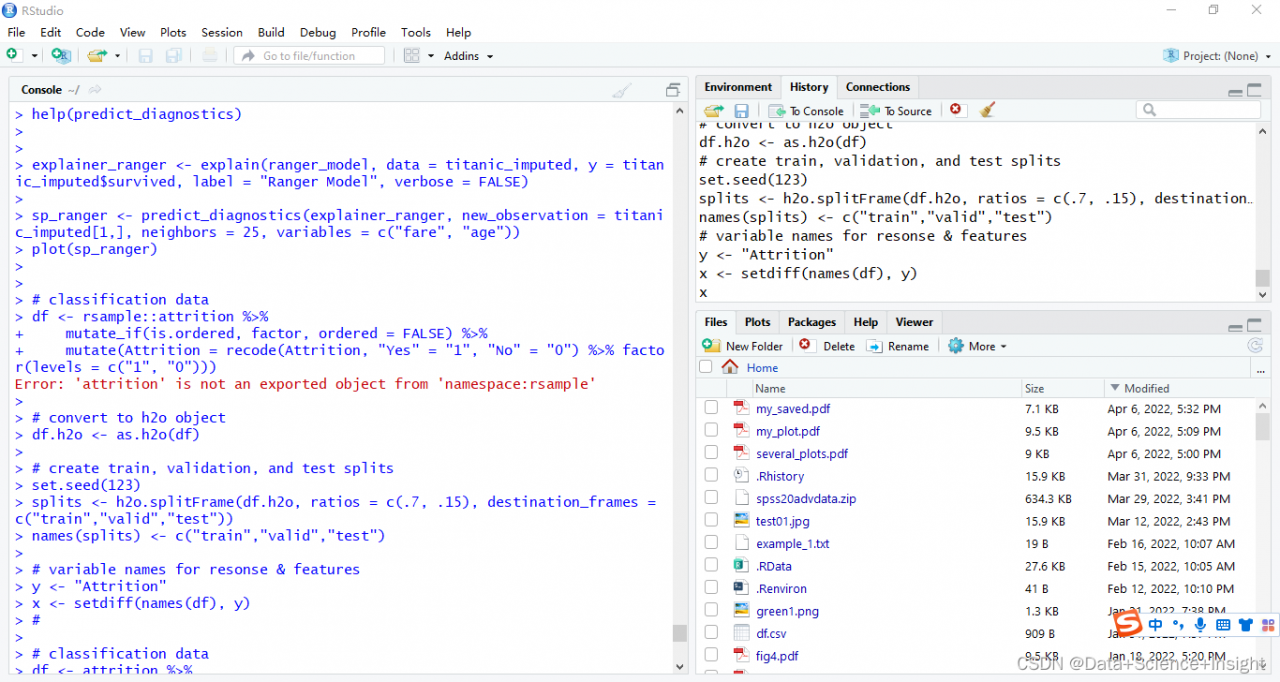# Error in value[[3L]](cond) : Package ‘rhdf5‘ version 2.36.0 cannot be unloaded:

``````library(rhdf5)
Error in value[[3L]](cond) : Package 'rhdf5' version 2.36.0 cannot be unloaded:Error in unloadNamespace(package) : namespace 'rhdf5' is imported by 'HDF5Array', 'MOFA2' so cannot be unloaded
``````

This is caused by importing packages from two places

Solution:

``````unloadNamespace('MOFA2')
library(rhdf5)
``````

success

# [Solved] Error: package or namespace load failed for ‘ggplot2’ in loadNamespace(i, c(lib.loc, .libPaths()), v

Error Messages:
> library(ggplot2)
Error: package or namespace load failed for ‘ggplot2’ in loadNamespace(i, c(lib.loc, .libPaths()), versionCheck = vI[[i]]):

Loaded namespace ‘ellipsis’ 0.3.1, but what is needed is >= 0.3.2

Solution:
In Rstudio, find the packages module, remove the package that reported the error and then re-install it using install.packages for the corresponding installation.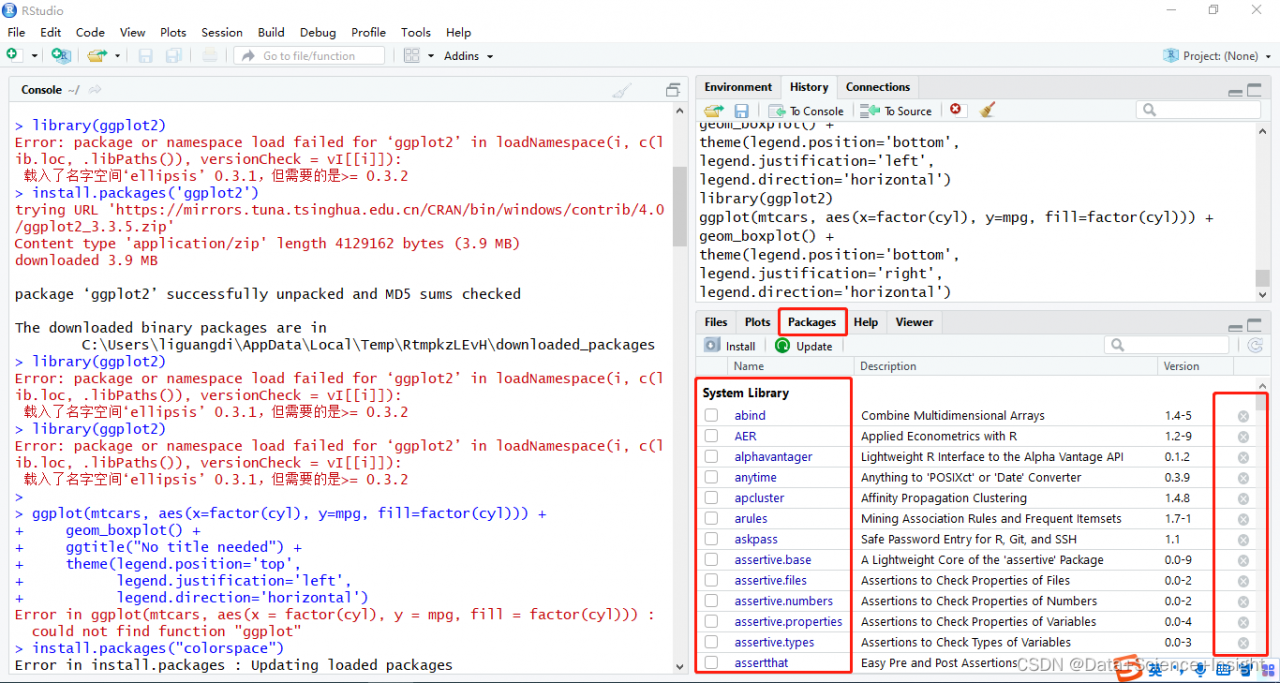# R Language: How to Solve featureplot function Error

This kind of error occurs when you run the featureplot function and want to generate a scatter diagram using ellipses, but the image cannot be generated due to the lack of ellipsse package. The error code is

``````Error in grid.Call.graphics(C_downviewport, name\$name, strict) :

Solution: only install the ellipse package, not the library, and then rerun the previous featureplot function.

``install.packages("ellipse")``

# [Solved] Linux R Pack Error: cram/cram_io.c:61:10: fatal error: lzma.h: No such file or directory

Error message

Operating system: Ubuntu 20.04 64 bit

An error occurred while installing rhtslib package. Error message:

``````> BiocManager::install("Rhtslib")
cram/cram_io.c:61:10: fatal error: lzma.h: No such file or directory
61 | #include <lzma.h>
|          ^~~~~~~~
compilation terminated.
make: *** [Makefile.Rhtslib:128: cram/cram_io.o] Error 1
make: Leaving directory '/tmp/Rtmp428cj4/R.INSTALL14a4e8febbab/Rhtslib/src/htslib-1.7'
make: *** [Makevars.common:24: htslib] Error 2
ERROR: compilation failed for package ‘Rhtslib’
* removing ‘/usr/local/lib/R/site-library/Rhtslib’

Solution:

Generally, the compilation fails due to the lack of packages in the system. Solve such problems by installing libbz2 dev, zlib1g Dev and liblzma dev:

``````sudo apt-get install libbz2-dev
sudo apt-get install zlib1g-dev
sudo apt-get install liblzma-dev``````

# [Solved] sbatch: error: Batch script contains DOS line breaks (\r\n)

When using the school HPC cluster, it is found that the batch task written by win10 reports an error, which can be solved with dos2unix.

Bugs and Solutions

This problem is due to the difference between UNIX and DOS blank line format. Just use code conversion
errors are reported as follows:

``````sbatch: error: Batch script contains DOS line breaks (\r\n)
sbatch: error: instead of expected UNIX line breaks (\n).
``````

Enter dos2unix:

``````dos2unix yourfilename
``````